# SOF - IMO PDF Sample Papers for Class 1

Class 1 sample paper & practice questions for International Mathematics Olympiad (IMO) level 1 are given below. Syllabus for level 1 is also mentioned for these exams. You can refer these sample paper & quiz for preparing for the exam.#### Resources:

##### Sample Questions from Olympiad Success:
 Q.1 Q.2 Q.3 Q.4 Q.5 Q.6 Q.7 Q.8 Q.9 Q.10
 Q.1 Find the two missing numbers in the given series.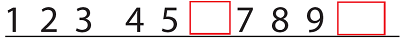a) 7, 45 b) 4, 5 c) 6, 10 d) 8, 6
 Q.2 Measure the length of bar.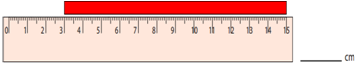a) 12 cm b) 15 cm c) 13 cm d) 11 cm
 Q.3 Fill in the blank: 89 metres = ___ centimetres. a) 8900 b) 1000 c) 100 d) 10000
 Q.4 Find the correct clock which shows the time to be 11:37. a)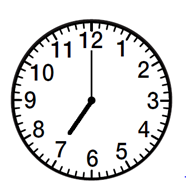b)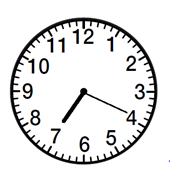c)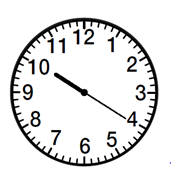d)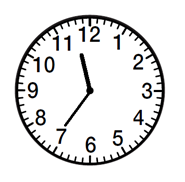Q.5 Which is the highest two-digit number? a) 11 b) 37 c) 28 d) 39
 Q.6 Jyoti is 168 cm tall and Lalita is 12 cm taller than Jyoti. How tall is Lalita? a) 170 cm b) 175 cm c) 185 cm d) 180 cm
 Q.7 Count the circles, write the numbers and find the sum.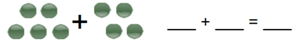a) 4 + 5 = 9 b) 4 + 4 = 8 c) 5 + 4 = 9 d) 5 + 4 = 10
 Q.8 What is the numeral of thirty seven? a) 95 b) 38 c) 58 d) 37
 Q.9 Write the time for the given clock.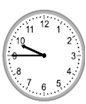a) 10:45 b) 9:45 c) 9:50 d) 10:40
 Q.10 Measure the object using blocks.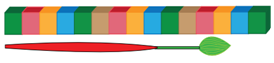a) 12 b) 15 c) 14 d) 13Sample PDF of SOF - International Mathematics Olympiad (IMO) PDF Sample Papers for Class 1:

 Q.1 )c Q.2 )a Q.3 )a Q.4 )d Q.5 )d Q.6 )d Q.7 )c Q.8 )d Q.9 )b Q.10 )d

Q.1 : c | Q.2 : a | Q.3 : a | Q.4 : d | Q.5 : d | Q.6 : d | Q.7 : c | Q.8 : d | Q.9 : b | Q.10 : d